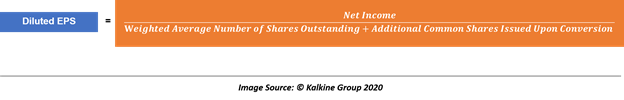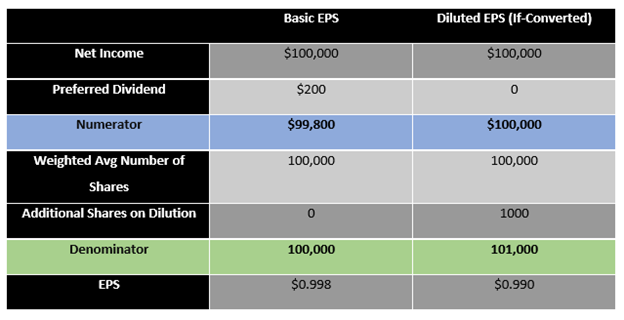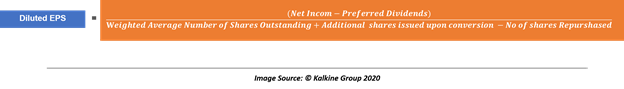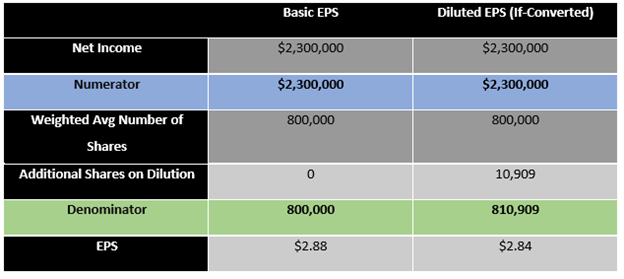Tue, September 21, 2021

# Diluted Earnings per Share

• Updated on

Diluted earnings per share is a type of earnings per share that is calculated considering all the convertible instruments such as convertible preferred shares, convertible debt, stock options, and right issues. Ideally, if a company does not have any convertible instrument outstanding, its basic earnings per share (or EPS) should be equal to its diluted earnings per share.

By definition, the diluted EPS of a company is always less or equal to the basic EPS; however, if a company has anti-dilutive instruments, the diluted EPS could be higher than the basic EPS. Under the financial reporting standards, i.e., IFRS and US GAAP, companies are required to disclose both basic and diluted EPS on the income statement.

## Calculating Diluted EPS for Various Dilutive Instruments

A majority of formulae used to calculate the diluted EPS of a company work on an if-converted method, that is, based on what EPS would have been of the convertible instrument under consideration if it is converted at the beginning of the period.

• Diluted EPS for Convertible Preferred Stocks

As suggested above, the calculation of diluted EPS when a company has convertible preferred stocks outstanding involves an if-converted method and assumes that the convertible preferred stocks had been converted at the beginning of the period.

If we use the if-converted method and assume that the convertible preferred stocks have been converted at the beginning of a period, it will leave two effects:

1. The convertible preferred stocks will no longer be outstanding; instead, additional common stocks will be outstanding (leading to a dilution in basic EPS).
2. If such a conversion took place, the company would not be paying any preferred dividends.

Therefore, if the above two effects take place, the number of shares outstanding would increase along with the net income available for distribution among common shareholders.

Thus, diluted EPS for such instruments could be calculated as below:For example, suppose a company had a net income of \$100,000 and an average of 100,000 shares of common stock. Let’s assume for simplicity that the company pays a preferred dividend of \$1 on 200 preferred shares (convertible into five common shares)

Under the if-converted method:• Diluted EPS for Convertible Debt Outstanding

When a company has convertible debt outstanding, the diluted EPS calculation once again considers the if-converted method, and diluted EPS is calculated as if the convertible debt outstanding had been converted at the beginning of the period.

So, if a company’s convertible debt is converted at the beginning of the period, it will leave two effects:

1. The company’s debt would no longer exist, and it will not pay any interest on the converted debt. Therefore, the net income available for common shareholders will increase by the after-tax amount of interest expense on debt converted.
2. Additional common shares will be issued and outstanding.

Thus, the calculation of diluted EPS under the if-converted method for convertible debt outstanding would be as below:

For example, suppose a company has a net income of \$750,000 and a weighted average shares of 690,000 at the end of a period. For simplicity, let us assume that the company has only one dilutive debt of \$50,000 @ 6 per cent of convertible debt, convertible into 10,000 shares. (Tax rate = 30 per cent)

Under the if-converted method:

• Diluted EPS For Stock Options, Warrants, Or Equivalent Outstanding

Just like the other two cases, if a company has stock options, warrants, or equivalent outstanding, the calculation again involves the if-converted method with a slight modification.

It is assumed that if a company has these dilutive instruments, it will convert the same at the beginning of the period and use the proceeds for repurchasing as many common shares as possible at the average market price.

The weighted average number of shares thus increases by the difference of outstanding shares minus the number of shares repurchased. Once a company dilutes such instruments, it will leave two effects:

1. The company will receive cash upon exercising.
2. The company is assumed to use the proceeds to repurchase shares from the market during the period at a weighted average market price.

Thus, the calculation of diluted EPS under the if-converted method for such instruments is as below:For example, suppose a company has a net income of \$2,300,000 and a weighted average of 800,000 shares at the end of a period. let us assume that the company has only one dilutive stock options @ 30,000 options at an exercise price of \$35. Also, let us assume that the market price of the company’s shares averaged \$55 per share.

Under the if-converted method:## Issues With Diluted EPS

The only issue which diluted EPS faces is the impact of anti-dilutive convertible securities as their inclusion into the calculation of diluted EPS would lead to a higher diluted EPS as compared to the basic EPS.

Under major financial reporting standards such as IFRS and US GAAP, anti-dilutive securities are not included in the calculation of diluted EPS. Ideally, diluted EPS should reflect the maximum dilution impact on basic EPS.

Furthermore, EPS is sensitive to change in the net income and a change in the number of outstanding shares, i.e., an increase in the net income or a decline in the number of outstanding shares or a combination of both could impact the y-o-y change in EPS.

Thus, it becomes paramount to understand and analyse EPS in the cross-sectional and time-series setting as a higher EPS does not necessarily reflect the true profitability on a standalone basis.